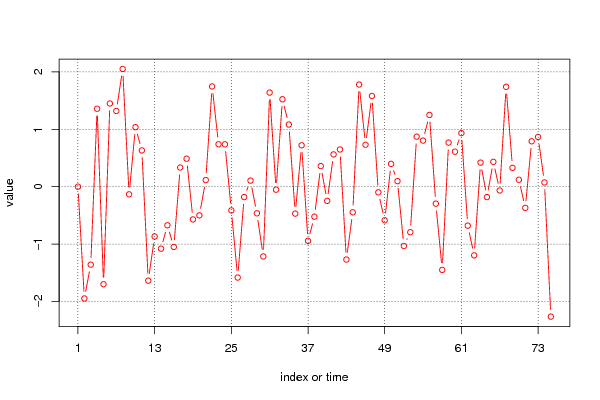## Free Statistics

of Irreproducible Research!

Author's title
Author*The author of this computation has been verified*
R Software Modulerwasp_univariatedataseries.wasp
Title produced by softwareUnivariate Data Series
Date of computationFri, 25 Nov 2011 05:19:22 -0500
Cite this page as followsStatistical Computations at FreeStatistics.org, Office for Research Development and Education, URL https://freestatistics.org/blog/index.php?v=date/2011/Nov/25/t13222163895ag77l99yupon4b.htm/, Retrieved Fri, 02 Jun 2023 08:53:04 +0000
Statistical Computations at FreeStatistics.org, Office for Research Development and Education, URL https://freestatistics.org/blog/index.php?pk=147279, Retrieved Fri, 02 Jun 2023 08:53:04 +0000
QR Codes:Original text written by user:
IsPrivate?No (this computation is public)
User-defined keywords
Estimated Impact128
Family? (F = Feedback message, R = changed R code, M = changed R Module, P = changed Parameters, D = changed Data)
-       [Univariate Data Series] [Plot and Describe...] [2011-11-25 10:19:22] [f59ea4acf51788e47c4ec521df29536b] [Current]
- RM      [Univariate Data Series] [] [2011-11-28 17:55:42] [74be16979710d4c4e7c6647856088456]
- R       [Univariate Data Series] [] [2011-11-28 17:56:06] [74be16979710d4c4e7c6647856088456]
Feedback Forum

Post a new message
Dataseries X:
0
-1.948342641
-1.359385149
1.357954964
-1.700694682
1.449969008
1.319038156
2.051491606
-0.133535063
1.037244962
0.631422616
-1.637925413
-0.867991129
-1.07958031
-0.672654982
-1.05064355
0.333390996
0.487322267
-0.570817054
-0.499534007
0.115264994
1.74691902
0.739021637
0.738226066
-0.412999274
-1.583363411
-0.181363817
0.105418964
-0.463545968
-1.216121966
1.640603862
-0.052871366
1.523685005
1.083344688
-0.469412704
0.721118803
-0.943920679
-0.52258191
0.359348877
-0.247868669
0.562507598
0.648148736
-1.270108506
-0.447026313
1.78025952
0.731000414
1.581054158
-0.098455366
-0.585819713
0.397301065
0.096307367
-1.033663768
-0.793451127
0.871673984
0.803259829
1.249946587
-0.295993913
-1.450053283
0.767875716
0.609240092
0.933296633
-0.679282808
-1.196592669
0.418815652
-0.181297827
0.432789884
-0.06594299
1.739802997
0.328406732
0.120753615
-0.369205393
0.79144574
0.866899133
0.074081225
-2.264885838

 Summary of computational transaction Raw Input view raw input (R code) Raw Output view raw output of R engine Computing time 1 seconds R Server 'Gwilym Jenkins' @ jenkins.wessa.net

\begin{tabular}{lllllllll}
\hline
Summary of computational transaction \tabularnewline
Raw Input & view raw input (R code)  \tabularnewline
Raw Output & view raw output of R engine  \tabularnewline
Computing time & 1 seconds \tabularnewline
R Server & 'Gwilym Jenkins' @ jenkins.wessa.net \tabularnewline
\hline
\end{tabular}
%Source: https://freestatistics.org/blog/index.php?pk=147279&T=0

[TABLE]
[ROW][C]Summary of computational transaction[/C][/ROW]
[ROW][C]Raw Input[/C][C]view raw input (R code) [/C][/ROW]
[ROW][C]Raw Output[/C][C]view raw output of R engine [/C][/ROW]
[ROW][C]Computing time[/C][C]1 seconds[/C][/ROW]
[ROW][C]R Server[/C][C]'Gwilym Jenkins' @ jenkins.wessa.net[/C][/ROW]
[/TABLE]
Source: https://freestatistics.org/blog/index.php?pk=147279&T=0

Globally Unique Identifier (entire table): ba.freestatistics.org/blog/index.php?pk=147279&T=0

As an alternative you can also use a QR Code:

The GUIDs for individual cells are displayed in the table below:

 Summary of computational transaction Raw Input view raw input (R code) Raw Output view raw output of R engine Computing time 1 seconds R Server 'Gwilym Jenkins' @ jenkins.wessa.net

 Univariate Dataseries Name of dataseries Source Description Number of observations 75

\begin{tabular}{lllllllll}
\hline
Univariate Dataseries \tabularnewline
Name of dataseries &  \tabularnewline
Source &  \tabularnewline
Description &  \tabularnewline
Number of observations & 75 \tabularnewline
\hline
\end{tabular}
%Source: https://freestatistics.org/blog/index.php?pk=147279&T=1

[TABLE]
[ROW][C]Univariate Dataseries[/C][/ROW]
[ROW][C]Name of dataseries[/C][C][/C][/ROW]
[ROW][C]Source[/C][C][/C][/ROW]
[ROW][C]Description[/C][C][/C][/ROW]
[ROW][C]Number of observations[/C][C]75[/C][/ROW]
[/TABLE]
Source: https://freestatistics.org/blog/index.php?pk=147279&T=1

Globally Unique Identifier (entire table): ba.freestatistics.org/blog/index.php?pk=147279&T=1

As an alternative you can also use a QR Code:

The GUIDs for individual cells are displayed in the table below:

 Univariate Dataseries Name of dataseries Source Description Number of observations 75PNG link Postscript link PDF link

Parameters (Session):
par4 = 12 ;
Parameters (R input):
par1 = ; par2 = ; par3 = ; par4 = 12 ;
R code (references can be found in the software module):
if (par4 != 'No season') {par4 <- as.numeric(par4)if (par4 < 4) par4 <- 12}summary(x)n <- length(x)bitmap(file='test1.png')if (par4=='No season') {plot(x,col=2,type='b',main=main,xlab=xlab,ylab=ylab,xaxt='n')axis(1,at=seq(1,n,10))}if (par4!='No season') {plot(x,col=2,type='b',main=main,xlab=xlab,ylab=ylab,xaxt='n')axis(1,at=seq(1,n,par4))grid(nx=0,ny=NULL,col='black')abline(v=seq(1,n,par4),col='black',lty='dotted')}dev.off()load(file='createtable')a<-table.start()a<-table.row.start(a)a<-table.element(a,'Univariate Dataseries',2,TRUE)a<-table.row.end(a)a<-table.row.start(a)a<-table.element(a,'Name of dataseries',header=TRUE)a<-table.element(a,par1)a<-table.row.end(a)a<-table.row.start(a)a<-table.element(a,'Source',header=TRUE)a<-table.element(a,par2)a<-table.row.end(a)a<-table.row.start(a)a<-table.element(a,'Description',header=TRUE)a<-table.element(a,par3)a<-table.row.end(a)a<-table.row.start(a)a<-table.element(a,'Number of observations',header=TRUE)a<-table.element(a,length(x))a<-table.row.end(a)a<-table.end(a)table.save(a,file='mytable.tab')